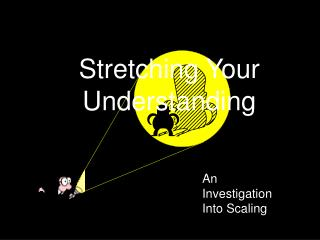DownloadDownload PresentationDownload Presentation- - - - - - - - - - - - - - - - - - - - - - - - - - - E N D - - - - - - - - - - - - - - - - - - - - - - - - - - -
##### Presentation Transcript

1. Stretching Your Understanding An Investigation Into Scaling

2. Wall Guy

4. Shadow Guy How many times taller is the shadow than the guy? Let’s call this number the scaling factor for height.

5. So it seems that the shadow is 3 times as tall as the guy. That is, the scaling factor for height between the guy and the shadow is 3. Often, we say that the guy’s height has been scaled up by a factor of 3.

6. Suppose we measure and determine that the guy is 2 meters tall and shadow is 6 meters tall. Shadow Guy

7. Suppose we measure and determine that the guy is 2 meters tall and shadow is 6 meters tall. Shadow 6m Guy 2m

8. Suppose we measure and determine that the guy is 2 meters tall and shadow is 6 meters tall. Shadow 6m Guy 2m How might we determine the scaling factor mathematically?

9. shadow’s height guy’s height So, the scaling factor =

10. shadow’s height guy’s height So, the scaling factor = =

11. shadow’s height guy’s height 6m 2m So, the scaling factor = =

12. shadow’s height guy’s height 6m 2m So, the scaling factor = = = 3

15. Height and width scaled equally Unscaled Height scaled more than width Width scaled more than height

16. Similar Figures Height and width scaled equally Unscaled Height scaled more than width Width scaled more than height

17. Not Similar Figures Height and width scaled equally Unscaled Height scaled more than width Width scaled more than height

18. What to remember: How to calculate the scaling factor: Dimension of Big Object Scaling factor = Dimension of Small Object What similarity means in this context: All dimensions are scaled by the same factor

19. What you will do: Part I: Find the scaling factors of the toy car for each dimension (height, length, and width)

20. What you will do: Part I: Find the scaling factors of the toy car for each dimension (height, length, and width) Part II a: Find the area of the parking space in which the toy car would park as well as that of the real car. Find the scaling factor for area. Using string and tape, mark out this area on the floor.

21. What you will do: Part II b: Make a box for your toy car by constructing a net using the graph paper provided. Find the scaling factor for volume.

22. Remember Similarity? Similar or not?

23. Remember Similarity? Similar or not?

24. Remember Similarity? Similar or not?

25. Remember Similarity? Similar or not?

26. Remember Similarity? Similar or not?

27. Remember Similarity? We call this self-similarity.

28. Now we have self-similarity on two scales.

29. Now we have self-similarity on two scales.

30. Now we have self-similarity on two scales.

31. Now we have self-similarity on three scales.

32. Now we have self-similarity on three scales.

33. Now we have self-similarity on three scales.

34. Now we have self-similarity on three scales.